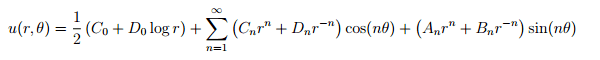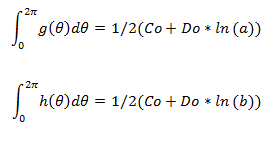# Fourier coefficients - dirichlet problem for annulus

• Dassinia

#### Dassinia

Hello

1. Homework Statement

Find the Fourrier coefficients in the annulus problem of the text.
uxx+uyy=0 in 0<a²<x²+y²<b²
u=g(θ) for x²+y²=a²
u=h(θ) for x²+y²=b²

## Homework Equations

The solution is## The Attempt at a Solution

I have the solutions but when I solved it for Co and Do I didn't get the same thing, but or all the other coefficients I got the right thing.

Here is the problem
To get Co and Do I did the integral on both sides from 0 to 2π to get rid of the sum.
So I ended up withBut the solution give a factor 1/π Before the integral of h and g , why ?
Thank you !

Try $$\int_0^{2\pi}u(a,\theta) \, d \theta$$ and see what you get. You'll have two sides, one is ##g## and the other is the long series you've posted.